# Introduction

The rationale of graph4lg package in R is to make easier the construction and analysis of genetic and landscape graphs in landscape genetic studies (hence the name graph4lg, meaning Graphs for Landscape Genetics). This package provides users with tools for:

• Landscape and genetic data processing
• Genetic graph construction and analysis
• Landscape graph construction and analysis
• Landscape and genetic graph comparisons

Each one of the included tutorials focuses on one of these points. This second tutorial will focus on genetic graph construction and analysis. It will describe the package functions allowing users to:

• Make preliminary analyses to choose a pruning method
• Construct genetic graphs with different pruning methods and link weighting
• Analyse genetic graphs (metrics, partition, plots, export)

## Data used in this tutorial

The package already includes genetic and spatial simulated data sets allowing users to discover its different functionalities. The first data set (data_simul) was simulated with CDPOP (Landguth and Cushman 2010) on a simulated landscape. It consists of 1500 individuals from 50 populations genotyped at 20 microsatellite loci. Individuals dispersed less when the cost-distance between populations was large. A landscape graph was created with Graphab (Foltête, Clauzel, and Vuidel 2012) whose nodes were the 50 simulated populations and the links were weighted by cost-distance values between populations. The project created with Graphab was included into the package such that the landscape graphs and the cost-distance matrix can be easily imported into the R environment.

Here, we also rely on a data set created only for the vignettes (data_tuto) and containing several objects created from the same data as that used to create data_simul:

data("data_tuto")

mat_dps <- data_tuto[]
mat_pg <- data_tuto[]
graph_ci <- data_tuto[]
dmc <- data_tuto[]
land_graph <- data_tuto[]
mat_ld <- data_tuto[]

# Preliminary analyses: diagnostic plots

A genetic graph is made of a set of nodes corresponding to sampled populations connected by a set of links between them. Usually, links are weighted by genetic distances between populations. A lot of different methods exist for constructing genetic graphs. They mainly differ in the way they conserve or remove links between population pairs, i.e. the way they prune the graph, and in the way links are weighted (which genetic distance?).

To choose a genetic distance and a pruning method for the genetic graph construction, we developed functions to perform preliminary analyses of the spatial pattern of genetic differentiation. Indeed, a genetic graph can be created in order to i) identify the direct dispersal paths between populations or to ii) select the set of population pairs to consider to infer landscape effects on dispersal. According to the use of a genetic graph and to the spatial pattern of genetic differentiation (type-I or type-IV pattern of IBD Van Strien, Holderegger, and Van Heck (2015)), the choice of a genetic distance and of a pruning method will not be the same.

Van Strien, Holderegger, and Van Heck (2015) computed the so-called distance of maximum correlation (DMC) as the distance between populations below which population pairs should be considered in order to maximise the correlation between landscape distance (geographical distance in their case, but applies similarly to cost-distance) and genetic distance. This distance threshold is computed by increasing iteratively the maximum distance between populations above which population pairs are not taken into account to compute the correlation. Thus, an increasing number of population pairs is considered in the inference. When the correlation coefficient between landscape distance and genetic distance reaches a maximum, the distance threshold considered is the DMC. When the DMC is equal to the maximum distance between populations, it means that an equilibrium established between gene flow and genetic drift at the scale of the study area. Conversely, when the DMC is lower than this maximum distance, it means that there is a “plateau” in the relationship between landscape distance and genetic distance because migration-drift equilibrium has not been reached yet at the scale considered. It can be due to recent modifications of the landscape which consistently reduced the connectivity in a previously connected context. In this case, graph pruning is needed to well infer landscape effect on dispersal. Similarly, genetic distances that do not assume this equilibrium should be used.

## Distance of maximum correlation

The function dist_max_corr calculates the DMC from two distance matrices. We need to specify the interval between two distance thresholds iteratively considered to select population pairs and compute the correlation coefficient.

dmc <- dist_max_corr(mat_gd = mat_dps, mat_ld = mat_ld,
interv = 500, pts_col = "black")

The dmc object is a list with 1) the DMC value, 2) a vector containing all the computed correlation coefficients, 3) a vector with all the distance thresholds tested and 4) a graphic object created with the ggplot2 package.

# DMC value
dmc[]
#>  4500
# Correlation coefficients
dmc[]
#>          NA 0.2986565 0.3154498 0.5188747 0.7059633 0.7559539 0.7850267
#>   0.7947691 0.8038470 0.7853646 0.7760106 0.7641339 0.7530264 0.7462445
#>  0.7386713 0.7333936 0.7305631 0.7226695 0.7137972 0.7110962 0.7041702
# Threshold distances tested
dmc[]
#>     500.00  1000.00  1500.00  2000.00  2500.00  3000.00  3500.00  4000.00
#>    4500.00  5000.00  5500.00  6000.00  6500.00  7000.00  7500.00  8000.00
#>   8500.00  9000.00  9500.00 10000.00 10230.05

The figure below represents the evolution of the correlation coefficient values when distance thresholds increase.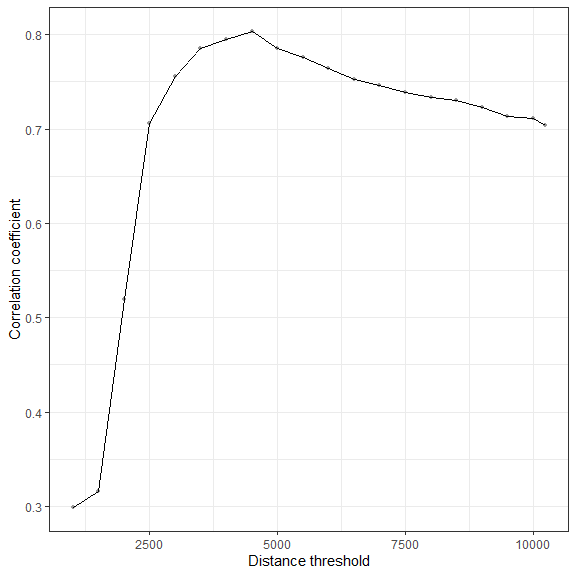## IBD pattern as a scatter plot

The function scatter_dist, on the other hand, allows users to visualise the relationship between two distance matrices by making a scatter plot. The shape of this relationship can be compared to the four different types of IBD patterns described by Hutchison and Templeton (1999) in order to characterise the spatial pattern of genetic differentiation.

For example:

scatter_dist(mat_gd = mat_dps, mat_ld = mat_ld,
pts_col = "black")
#> 1225 out of 1225 values were used.
#> geom_smooth() using formula 'y ~ x'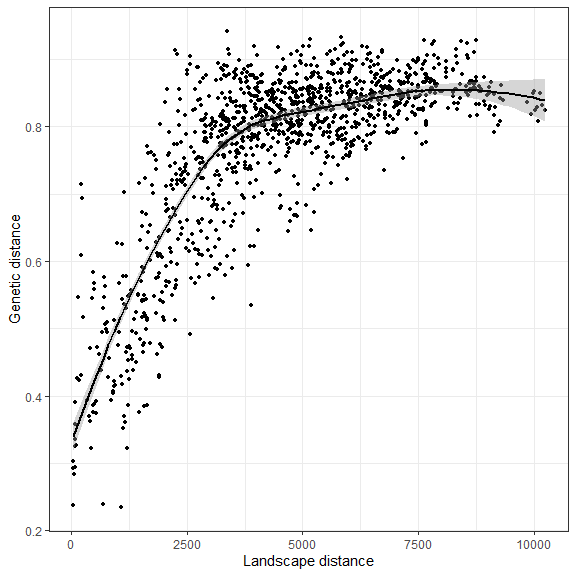In this particular case, we notice a type-IV pattern of isolation by distance with a “plateau” in the relationship between cost-distance and genetic-distance (DPS). Graph pruning will be needed to select the population pairs to include in the inference of landscape effects on dispersal.

# Genetic graph construction

Once the diagnostic plots have been created, users do have some indications to construct the genetic graphs. Pruning is especially needed when there is a “plateau” in the relationship between genetic distance and landscape distance. In the following section, we present the different pruning methods available.

## Pruning based on distance thresholds

To prune a graph whose links are weighted by distances, we can remove all the links associated to geographical or genetic distances larger (or lower) than a specific threshold distance. This distance can for example be equal to the maximum dispersal distance of an individual of the study species at the scale of its lifespan so that the resulting graph represents the direct dispersal paths of the species. It can also be equal to the DMC if the objective is to infer landscape effects on dispersal.

The function gen_graph_thr takes as arguments a distance matrix used to weight the links of the resulting graph (mat_w) and a distance matrix on which the “thresholding” is based (mat_thr). The selected links are selected according to the values of this latter matrix. The argument thr is the numerical value of the threshold distance. If mat_thr is not specified, mat_w is used by default for the thresholding. Lastly, we have to specify if the links to remove take larger or lower values than the threshold value.

# First compute the geographical distance between populations
mat_geo <- mat_geo_dist(data = pts_pop_simul,
ID = "ID", x = "x", y = "y",
crds_type = "proj")
#> Coordinates were treated as projected coordinates. Check whether
#>               it is the case.
# Reorder the matrix
mat_geo <- reorder_mat(mat_geo, order = row.names(mat_dps))

# Create the thresholded graph
graph_thr <- gen_graph_thr(mat_w = mat_dps, mat_thr = mat_geo,
thr = 12000, mode = "larger")
graph_thr
#> IGRAPH 387c323 UNW- 50 162 --
#> + attr: name (v/c), weight (e/n)
#> + edges from 387c323 (vertex names):
#>   1 --2  1 --4  1 --5  1 --6  1 --9  10--12 10--20 10--21 10--8  11--12
#>  11--16 11--18 11--20 11--4  11--5  11--8  11--9  12--18 12--20 12--21
#>  12--8  13--14 13--15 13--16 13--17 13--2  13--22 13--5  13--6  13--7
#>  14--15 14--16 14--17 14--22 14--5  14--6  15--16 15--17 15--22 15--5
#>  15--9  16--17 16--18 16--22 16--26 16--4  16--5  16--9  17--19 17--22
#>  17--23 17--27 17--28 17--6  18--20 18--21 18--24 18--25 18--26 18--8
#>  18--9  19--23 19--27 19--7  2 --4  2 --5  2 --6  2 --9  20--21 20--24
#>  20--25 20--26 20--8  21--24 21--29 22--26 22--28 22--30 23--27 23--28
#> + ... omitted several edges

The function returns a graph in the form of an igraph object, which is consequently compatible with all functions from igraph package (Csardi and Nepusz 2006), one of the most used R package to create and analyse graphs (together with sna and networks). In the latter example, the graph has 50 nodes and 162 links when we prune it using a 12-km distance threshold. Its links are weighted with the values of the mat_dps matrix.

## Pruning based on topological constraints

A graph can be pruned according to a topological criterion. The function gen_graph_topo can use 5 different criteria. As with the previous function, topological criteria are applied by considering the distance values of the mat_topo matrix, but the links are weighted with the values of the mat_w matrix (except when mat_topo is not specified, cf. previous section).

Gabriel graph: in the created graph, two nodes are connected by a link if, when we draw a circle whose center is set at the middle of the segment linking them and whose radius is equal to half the length of this segment, there is no other node inside the circle. In mathematical terms, it means that there is a segment between $$x$$ and $$y$$ if and only if for every other point $$z$$, we have: $$d_{xy}\leq \sqrt{d_{xz}^{2}+d_{yz}^{2}}$$. We can compute such a graph from geographical distances (Gabriel and Sokal 1969) (graph_gab_geo below) but also, less commonly, from genetic distances (Naujokaitis-Lewis et al. 2013) (graph_gab_gen below). In the latter case, it is to some extent as if Pythagoras’s theorem was applied to genetic distances, which has already been done by Naujokaitis-Lewis et al. (2013).

graph_gab_geo <- gen_graph_topo(mat_w = mat_dps, mat_topo = mat_geo,
topo = "gabriel")
graph_gab_geo
#> IGRAPH 38d0d1d UNW- 50 98 --
#> + attr: name (v/c), weight (e/n)
#> + edges from 38d0d1d (vertex names):
#>   1 --2  1 --5  10--12 10--21 11--12 11--16 11--18 11--8  11--9  12--20
#>  12--8  13--14 13--17 13--5  13--6  14--15 14--17 14--5  15--16 15--22
#>  15--5  16--18 16--22 16--9  17--19 17--23 17--27 17--28 18--20 18--25
#>  19--23 19--7  2 --6  20--21 20--24 20--25 21--24 21--29 22--26 22--28
#>  23--27 24--25 24--29 24--31 25--26 25--31 25--32 26--30 27--28 27--33
#>  28--30 28--34 28--36 29--31 29--37 3 --7  30--32 30--34 31--32 31--35
#>  31--37 32--34 32--35 33--36 33--42 34--36 34--44 35--37 35--39 35--45
#>  36--40 37--38 37--39 37--41 38--41 38--43 39--41 39--49 4 --5  4 --9
#> + ... omitted several edges
graph_gab_gen <- gen_graph_topo(mat_w = mat_dps, mat_topo = mat_dps,
topo = "gabriel")

Minimum Spanning Tree (MST): it creates a minimum spanning tree, i.e a graph in which every node is connected by a link to at least another node and whose total link weight is minimum. By definition, its number of links is equal to the number of nodes - 1.

graph_mst <- gen_graph_topo(mat_w = mat_dps, mat_topo = mat_dps,
topo = "mst")
graph_mst
#> IGRAPH 3923378 UNW- 50 49 --
#> + attr: name (v/c), weight (e/n)
#> + edges from 3923378 (vertex names):
#>   1 --2  1 --4  10--8  11--12 11--18 11--20 11--4  12--8  13--14 13--6
#>  14--15 15--17 15--22 15--28 16--23 17--23 19--23 2 --7  20--25 21--24
#>  23--27 24--29 25--29 25--30 26--30 27--33 3 --6  3 --7  30--34 31--32
#>  32--34 32--35 32--37 36--40 37--38 38--43 39--43 4 --9  40--42 41--43
#>  41--49 42--48 43--45 43--47 44--45 44--48 46--47 48--50 5 --9

“Percolation” graph: the graph is created by removing iteratively some links, beginning with those with the highest weights until the graph breaks into more than one component. We conserve the link whose removal entails the creation of another component to obtain a connected graph. This method is also called the edge-thinning method (Urban et al. 2009). Such a method is linked to percolation theory (Rozenfeld et al. 2008). The function gen_graph_topo indicates the number of conserved links and the weight of the link whose removal disconnects the graph (maximum link weight of the created graph).

graph_percol <- gen_graph_topo(mat_w = mat_dps, mat_topo = mat_dps,
topo = "percol")
#> Number of conserved links : 325
#> Maximum weight of the conserved links : 0.7525

“k-nearest-neighbors” graph: it creates a graph in which every node is connected to its $$k$$-nearest neighbors according to the distance matrix mat_topo. Its links are weighted with values from mat_w. It means that if the distance between node $$i$$ and node $$j$$ is among the $$k$$-th smallest distances between node $$i$$ and the other nodes, there is a link between $$i$$ and $$j$$ in the graph. Therefore, a node can be connected to more than $$k$$ nodes because the nearest node to node $$j$$ is not necessarily among the $$k$$ nearest neighbors to node $$i$$. The function gen_graph_topo takes topo="knn" and k=x as arguments in that case. For example :

graph_k3 <- gen_graph_topo(mat_w = mat_dps, mat_topo = mat_dps,
topo = "knn", k = 3)

Complete graph: the function allows users to create a complete graph from a distance matrix. In that case, there is no pruning and, by definition, all population pairs are connected.

graph_comp <- gen_graph_topo(mat_w = mat_dps, mat_topo = mat_dps,
topo = "comp")

Finally, the function graph_plan creates a planar graph. However, this method relies upon a Voronoi triangulation that needs spatial coordinates as input. Hence, it is not part of the gen_graph_topo function. The function graph_plan can be used as following:

#> Coordinates were treated as projected coordinates. Check whether
#>               it is the case.
#> Coordinates were treated as projected coordinates. Check whether
#>               it is the case.
#> IGRAPH 39bfa0c UNW- 50 136 --
#> + attr: name (v/c), weight (e/n)
#> + edges from 39bfa0c (vertex names):
#>   1 --2  1 --3  1 --4  1 --5  1 --8  2 --3  2 --5  2 --6  2 --13 3 --6
#>  3 --7  3 --19 4 --5  4 --8  4 --9  4 --11 5 --9  5 --13 5 --14 5 --15
#>  5 --16 6 --7  6 --13 7 --13 7 --19 8 --10 8 --11 8 --12 9 --11 9 --16
#>  10--12 10--21 10--29 11--12 11--16 11--18 12--18 12--20 12--21 13--14
#>  13--17 13--19 14--15 14--17 15--16 15--17 15--22 16--18 16--22 16--26
#>  17--19 17--22 17--23 17--27 17--28 18--20 18--25 18--26 19--23 19--33
#>  20--21 20--24 20--25 21--24 21--29 22--26 22--28 22--30 23--27 23--33
#>  24--25 24--29 24--31 25--26 25--31 25--32 26--30 26--32 27--28 27--33
#> + ... omitted several edges

## Pruning based on the conditional independence principle

The last pruning method implemented by the graph4lg package is based upon the conditional independence principle. The function gen_graph_indep is largely inspired by the function popgraph created by R. Dyer (Dyer and Nason 2004), but does not need the package popgraph to function. Besides, as some calculations are performed with functions from the adegenet package (coded in C), it is faster than the original popgraph function. It is also more flexible than popgraph function given we can vary i) the way we compute genetic distances used to weight the links and to compute the covariance between populations, ii) the formula used to compute the covariance from squared distances or alternatively simple distances, iii) the statistical tolerance threshold, iv) the p-values adjustment and v) the returned objects created by the function. Without entering further into the details, here is an implementation example.

graph_ci <- gen_graph_indep(x = data_genind,
dist = "PCA",
cov = "sq",
adj = "holm")
graph_ci
#> IGRAPH 30129d7 UNW- 50 105 --
#> + attr: name (v/c), weight (e/n)
#> + edges from 30129d7 (vertex names):
#>   1 --2  1 --3  1 --4  1 --9  10--11 10--8  11--12 11--18 11--20 12--20
#>  12--25 12--8  13--14 13--15 13--22 13--6  14--15 14--17 14--19 14--27
#>  14--32 14--6  15--17 15--23 15--28 16--22 16--23 16--33 16--41 17--23
#>  17--33 18--19 18--20 18--9  19--23 19--27 2 --5  2 --7  20--4  20--5
#>  21--24 21--29 21--45 22--23 22--8  23--27 23--49 24--29 25--26 25--3
#>  25--30 25--32 25--37 26--3  26--30 26--34 26--39 26--44 26--8  27--33
#>  3 --31 3 --6  3 --7  30--34 30--7  31--32 31--35 31--36 32--34 32--35
#>  32--8  33--40 34--6  35--37 36--40 36--48 37--38 37--39 37--46 38--43
#> + ... omitted several edges

# Genetic graph analysis

Once the genetic graphs have been created, we can perform calculations from them, visualise and export them.

## Computing graph-theoretic metrics

First, we can compute graph-theoretic metrics at the node-level from graphs with the function compute_node_metric (that uses in part functions from igraph package in R). This function takes a graph object and a vector indicating the metrics to compute as arguments. Available metrics are:

• Degree ("deg"): number of links connected to each node
• Closeness centrality ("close"): number of links between a node and every other nodes in the graph, measured as the inverse of the average length of the shortest paths to/from the focal node to/from all the other nodes in the graph.
• Betweeness centrality ("btw"): number of times each node is a step on the shortest path from a node to another, when considering all possible combinations.
• Strength ("str"): sum of the weights of the links connected to a node
• Sum of inverse weights ("siw"): sum of the inverse weights of the links connected to a node
• Mean of inverse weights ("miw"): mean of the inverse weights of the links connected to a node

The two latter metrics, when applied to genetic graphs whose links are weighted by genetic distances, reflect how similar a population is from the others and has been shown to be correlated with the number of migrants going to/from this population (Koen, Bowman, and Wilson 2016).

Link weights can be considered or not in the computation (weight = TRUE or weight = FALSE).

When used, this function returns a data.frame with the values of the computed metrics for each node.

df_metric <- compute_node_metric(graph = graph_percol)
head(df_metric)

Metric values can then be associated with the nodes to which they correspond in the graph object itself. To that purpose, we use the function add_nodes_attr and give it as arguments:

• the name of the graph (which must have node names)(graph),
• (if input = "df") the name of the data.frame containing the values to add as node attributes (data),
• the name of the column in which node names are stored. It will be used to merge the graph node attribute table with the data.frame (index)
• the name of the columns to include as node attributes. If not specified, all columns are included (ìnclude="all"(by default) or include=c("metric1", "metric2", ...)).

For example, we can add the metrics from df_metric to the nodes of the graph graph_percol from which they were computed:

graph_percol <- add_nodes_attr(graph = graph_percol,
data = df_metric,
index = "ID",
include = "all")
graph_percol
#> IGRAPH 3938931 UNW- 50 325 --
#> + attr: name (v/c), deg (v/n), close (v/n), btw (v/n), str (v/n), siw
#> | (v/n), miw (v/n), weight (e/n)
#> + edges from 3938931 (vertex names):
#>   1 --11 1 --12 1 --18 1 --2  1 --20 1 --25 1 --3  1 --4  1 --5  1 --7
#>  1 --9  10--11 10--12 10--18 10--20 10--25 10--8  11--12 11--18 11--2
#>  11--20 11--25 11--26 11--30 11--34 11--4  11--5  11--8  11--9  12--18
#>  12--2  12--20 12--25 12--26 12--30 12--34 12--4  12--5  12--8  12--9
#>  13--14 13--15 13--16 13--17 13--19 13--22 13--23 13--27 13--28 13--3
#>  13--33 13--6  14--15 14--16 14--17 14--19 14--22 14--23 14--25 14--26
#>  14--27 14--28 14--3  14--30 14--33 14--34 14--40 14--6  14--7  15--16
#> + ... omitted several edges

The resulting object is the graph object of class igraph in which node attributes were added.

We can also associate metric values to the nodes of the igraph object by specifying the path to a shapefile layer whose attribute table contains a field with the graph node names. In this case, argument data is not used and we have to specify the path of the directory in which the shapefile layer is located (dir_path) and the root name of this layer (layer).

graph_percol <- add_nodes_attr(graph_percol,
input = "shp",
dir_path = system.file('extdata', package = 'graph4lg'),
layer = "patches",
index = "Id",
include = "Area")

## Graph partitioning

In a graph, some groups of nodes are more connected then they are connected to nodes from other groups. These groups form communities or modules. They can be identified through modularity analyses. The function compute_graph_modul makes possible this identification. Several algorithms can be used (argument algo): fast greedy (Clauset, Newman, and Moore 2004), louvain (Blondel et al. 2008), optimal (Brandes et al. 2008) and walktrap (Pons and Latapy 2006).

The number of created modules in each graph is adjustable but by default depends on the optimal value obtained when performing the modularity analysis (argument nb_modul).

Besides, the modularity calculation can take into account the way link weights represent the node interaction. When taken into account, the weight given to a link in the calculation can be:

• Considered as a distance (node_inter = "distance"): in that case, a link corresponding to a large distance between nodes is given a small weight in the analysis
• Considered as a similarity index (node_inter = "similarity"): in that case, a link corresponding to a large similarity between nodes is given a large weight in the analysis

For example:

df_modul <- compute_graph_modul(graph = graph_percol,
algo = "fast_greedy",
node_inter = "distance")

head(df_modul)
# Unique values of module ID
unique(df_modul\$module)
#>  "1" "2" "4" "3"

In this example, the optimal number of modules is 4. The returned object is a data.frame indicating the ID of the module to which each node pertains.

This information can also be added as a node attribute to the graph object.

graph_percol <- add_nodes_attr(graph = graph_percol,
input = "df",
data = df_modul,
index = "ID")

Now, graph_percol has many attributes which can be used in subsequent analyses. They can be displayed using the command igraph::get.vertex.attribute(graph_percol).

## Visual analysis

Visual representation of the graph on a map

Graphs, and especially spatial graphs, are particularly adapted to visual analyses. The function plot_graph_lg integrates functions from igraph and ggplot2 to represent graphs on a map.

Spatial graphs:

Most frequently, graphs are spatial and a table with population coordinates must be given as an argument. It must have exactly the same structure as the table given as an argument to mat_geo_dist (3 columns : ID, x, y). The visual representation can make visible the link weights by plotting the links with a width proportional to the weight (link_width = "w") or the inverse weight (link_width = "inv_w") of the links.

For example, with the graph graph_mst with mode="spatial":

p <- plot_graph_lg(graph = graph_mst,
mode = "spatial",
crds = pts_pop_simul,
p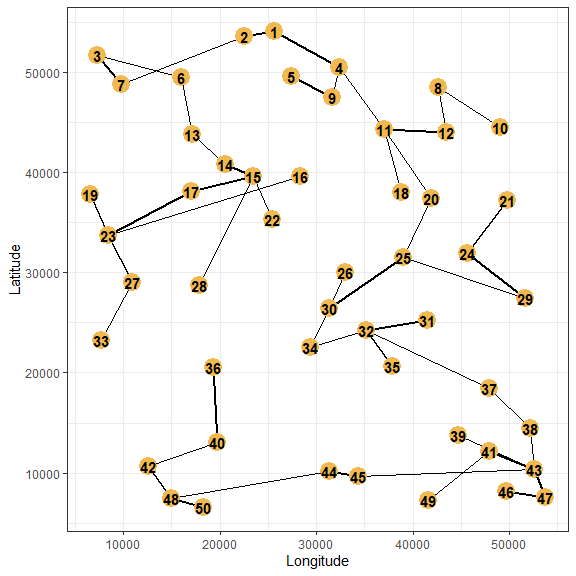Besides, the node size can be proportional to one of the node attributes, and their color can depend on the module of the node if a modularity analysis has been performed whose results were added to the graph object. For example, if we want to display both node metrics and modules for the graph graph_mst, the steps to follow are:

# Compute the metrics
df_metric_mst <- compute_node_metric(graph = graph_mst)

# Associate them to the graph
data = df_metric_mst,
index = "ID",
include = "all")
# Compute the modules
df_module_mst <- compute_graph_modul(graph = graph_mst,
algo = "fast_greedy",
node_inter = "distance")
# Associate them to the graph
data = df_module_mst,
index = "ID",
include = "all")

# Plot the graph
# Link width is inversely proportional to genetic distance
# Node size is proportional to MIW metric
# Node color depends on the node module

plot_graph_lg(graph = graph_mst,
mode = "spatial",
crds = pts_pop_simul,
node_size = "miw",
module = "module")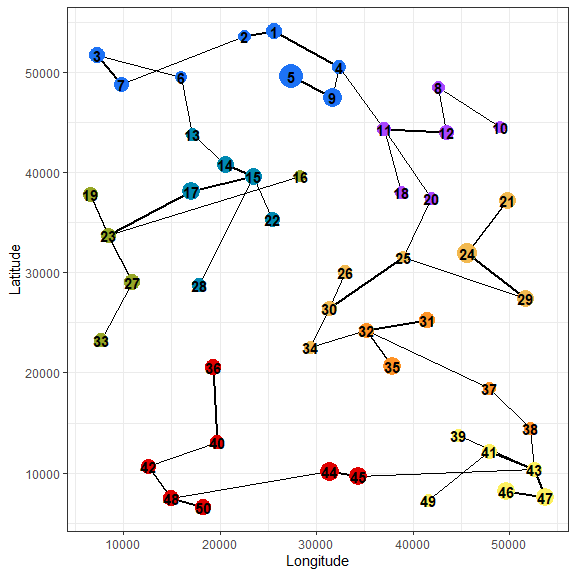Aspatial graph:

If the population spatial coordinates are not available, we can still display the graph on a two-dimensional plane. In that case, the node positions are computed with Fruchterman and Reingold (1991) algorithm to optimise the representation. This algorithm is based upon a principle of attraction-repulsion so that nodes with strong connections are close to each other, but not so close in order to avoid their overlap. This algorithm is used by the function plot_graph_lg when mode="aspatial". The way nodes interact can be specified and indicates if link weights correspond to distances or similarities. In the first case, links with large weights tend to separate nodes whereas in the latter case, large weights tend to attract nodes (node_inter = "distance" or node_inter = "similarity").

With the graph graph_mst, we obtain:

p <- plot_graph_lg(graph = graph_mst,
mode = "aspatial",
node_inter = "distance",
node_size = "miw",
module = "module")
p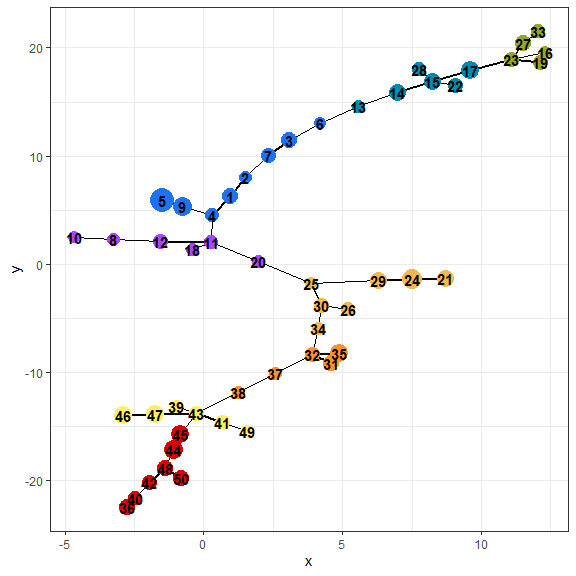Note that this aspatial representation can be useful even when spatial coordinates are available. Indeed, it indicates if neighbor populations from a geographical point of view are also neighbors in the aspatial representation only based on their genetic distances.

We see in that example that nodes from the same modules are direct neighbors in both the spatial and aspatial representations.

Representation of the links on a scatterplot

In landscape genetics, a graph is generally pruned from a distance matrix in which a set of distance values between population pairs or sample sites are chosen. This matrix is usually a genetic distance matrix. The relationship between these genetic distances and corresponding landscape distances (geographical or cost-distance) can be studied. When a scatterplot is created to do that (with the function scatter_dist), we can display the points corresponding to population pairs connected in the pruned graph in a different color. The function scatter_dist_g thereby allows users to understand the pruning and to assess its intensity.

In the following example, we can see that all connected population pairs from graph_gab_geo are separated by short landscape distances.

scatter_dist_g(mat_y = mat_dps ,
mat_x = mat_ld,
graph = graph_gab_geo)
#> geom_smooth() using formula 'y ~ x'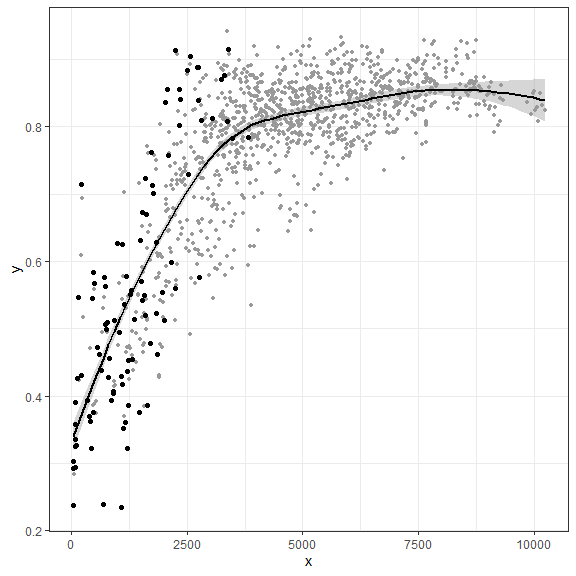Finally, in order to have further information about genetic differentiation patterns, we can create histograms depicting the link weight distribution with the function plot_hist_w.

p <- plot_w_hist(graph = graph_gab_gen)
p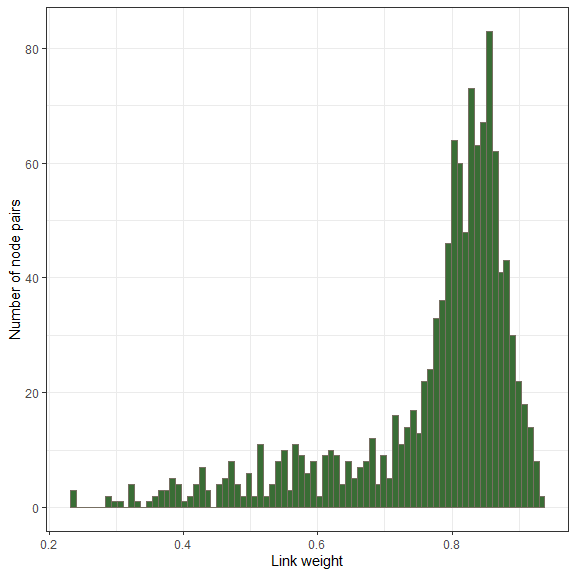## Export to shapefile layers

Even if the function plot_graph_lg enables to visualise a spatial graph on a geographical plane, it is often useful to confront the population and link locations to other types of spatial data. To that purpose, we can export the graph into shapefile layers in order to open them in a GIS. The graph nodes must have spatial coordinates. When exporting, we can choose to export only the node shapefile layer, the link shapefile layer or both. We can also export node attributes (metrics=TRUE). These attributes will be included in the attribute table of the exported node shapefile layer. For the links, the attribute table contains the weights associated to every link, if they exist.

The function graph_to_shp takes also as an argument the coordinates reference system (CRS) in which the point coordinates from the table are expressed. It will be the CRS of the created shapefile layers, expressed as an integer EPSG code. The last argument is the suffix given to the shapefile layer names beginning with “node” or “link”.

graph_to_shp(graph = graph_mst,
crds = pts_pop_simul,
mode = "both",
layer = "test_shp_mst",
dir_path = "wd",
metrics = TRUE,
crds_crs = 2154)

Shapefile layers are created in the working directory and can be imported into a GIS.

# Conclusion

In the next tutorial, we will present how to construct and analyse a landscape graph using Graphab with graph4lg.